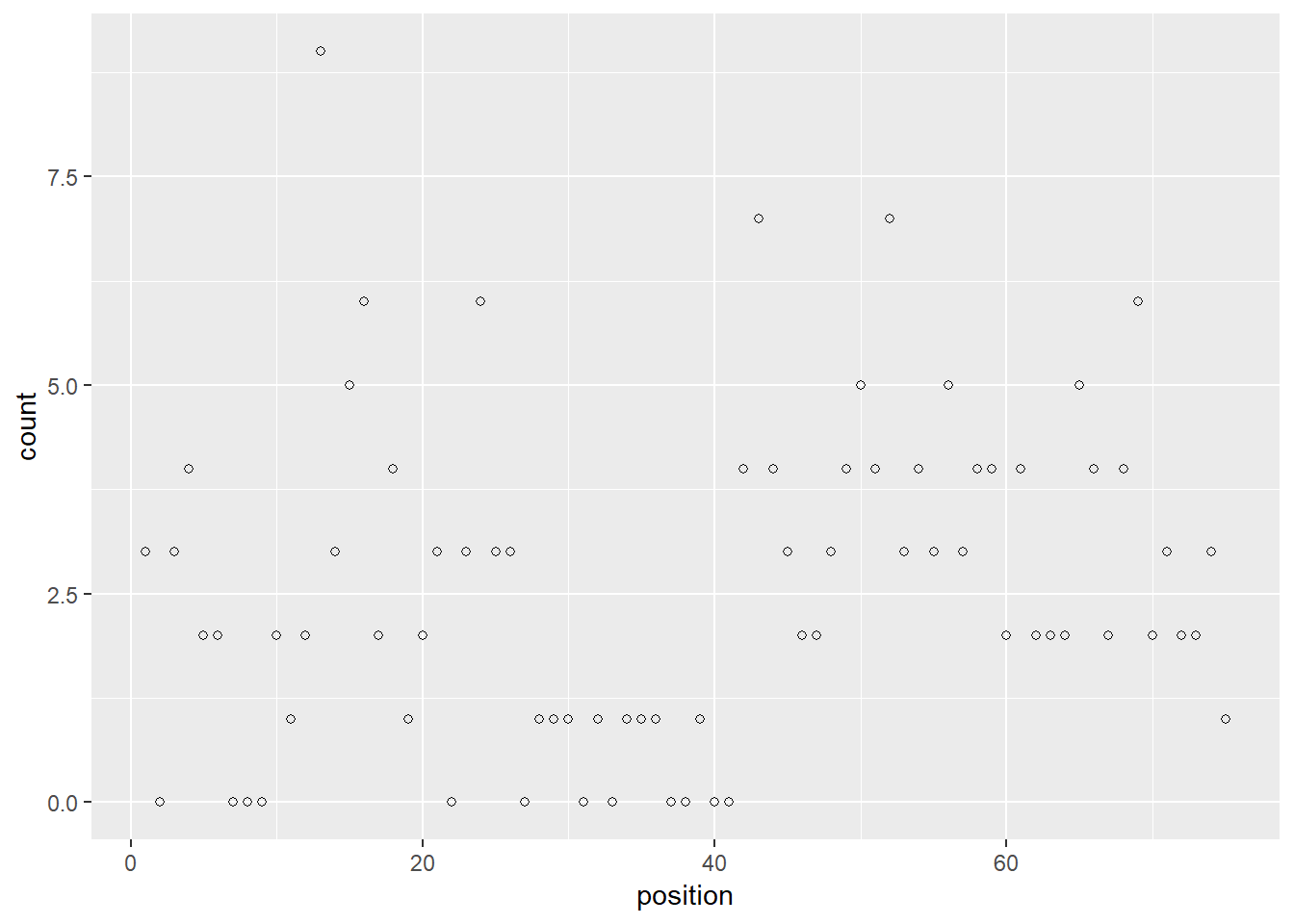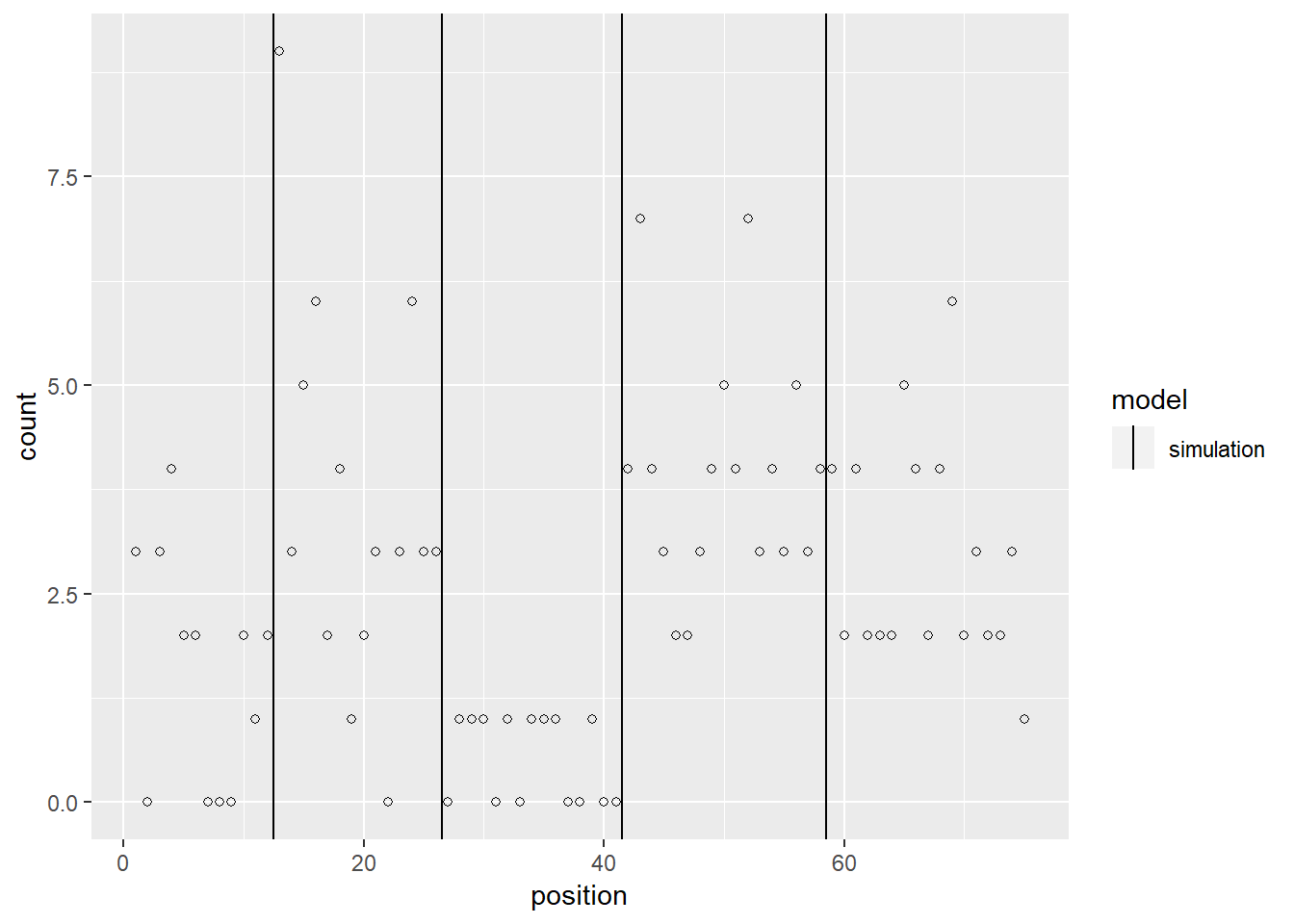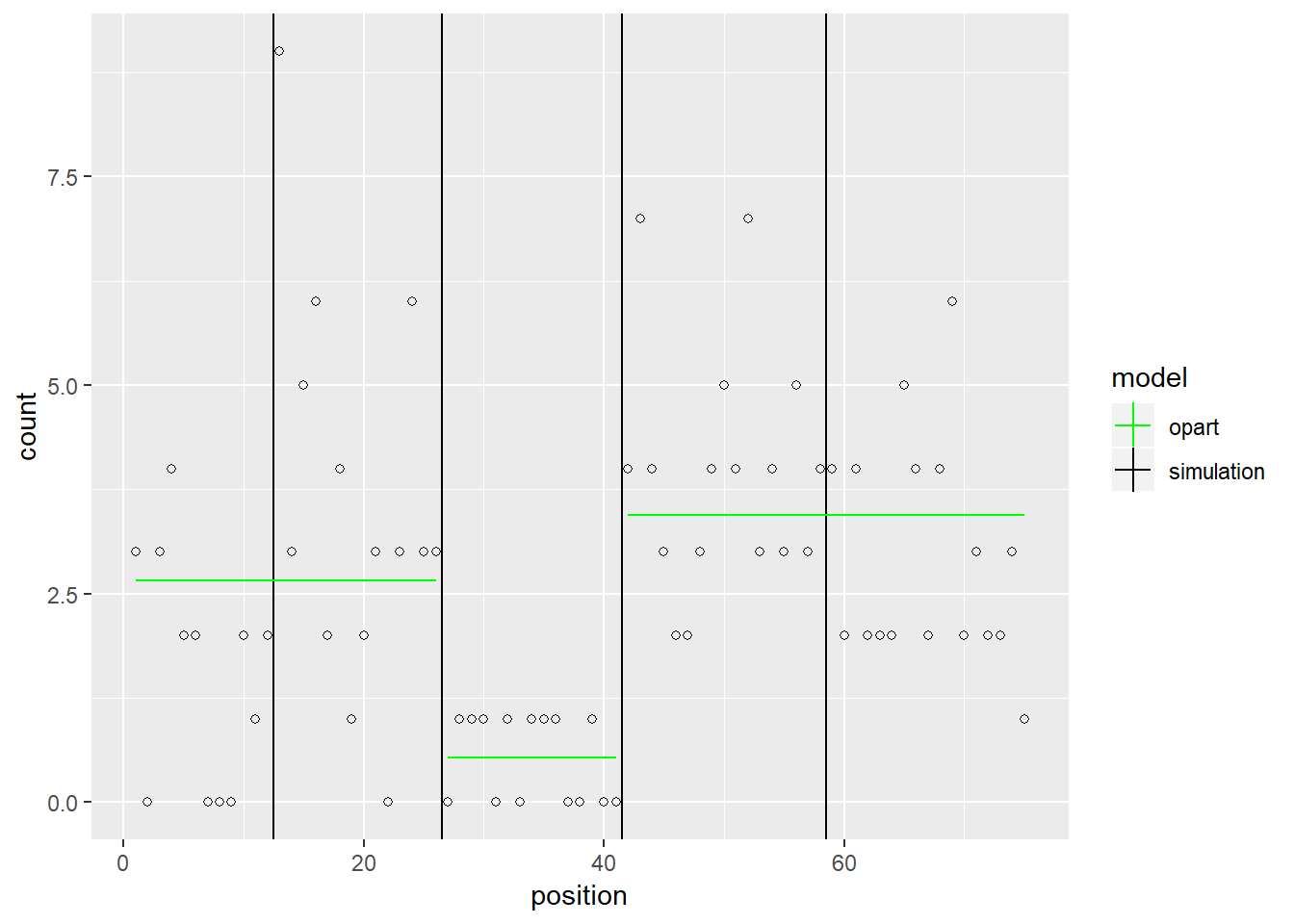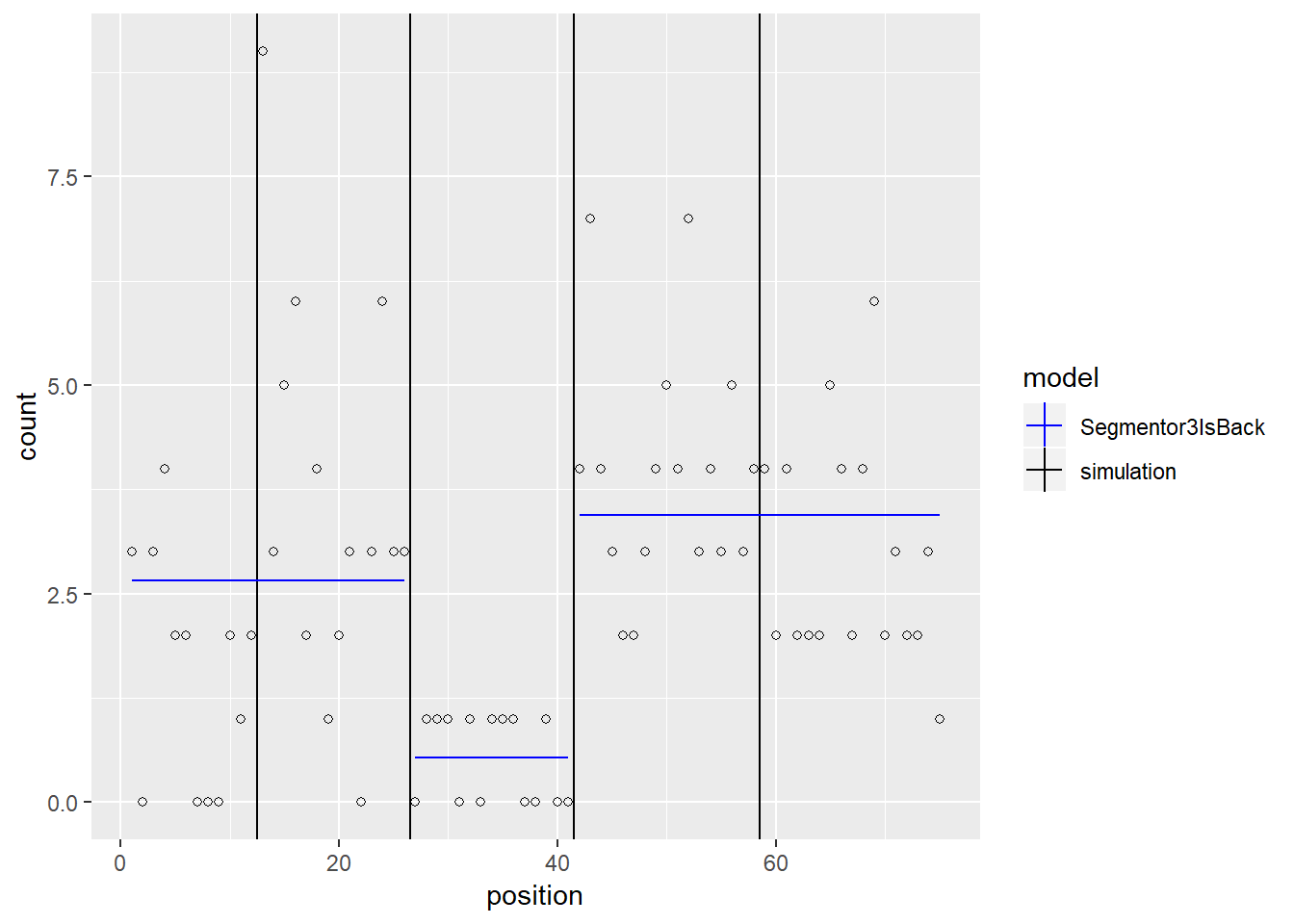### Optimal Partitioning with Poisson loss

This vignette introduces Poisson loss function in statistics and summarizes important results from Poisson distribution as well as functions available in this package for solving the standard optimal partitioning problem using Poisson loss as cost function.

### Introduction

In statistics, Poisson regression is a generalized linear model form of regression analysis used to model count data and contingency tables. Poisson regression assumes the response variable Y has a Poisson distribution, and assumes the logarithm of its expected value can be modeled by a linear combination of unknown parameters. A Poisson regression model is sometimes known as a log-linear model, especially when used to model contingency tables.

The formula for the Poisson probability mass function is:

$P(x, \lambda) = \frac{{e^{ - \lambda } \lambda ^x }}{{x!}}$

Where $$\lambda$$ is the shape parameter which indicates the average number of events in the given time interval and x is the input variable for which the probability is calculated. The values of x are independent and identically(iid) distributed.

### Maximum Likelihood Estimation

Maximum likelihood estimation (MLE) is an estimation method that allows to use a sample to estimate the parameters of the probability distribution that generated the sample.

For Poisson Distibution the parameter of interest is $$\lambda$$. If we draw a sequence $$X_{n}$$ of n independent observations from a Poisson Distribution then the probability mass function for each term of the sequence is given by (1). Therefore, the probability of observing this sequence $$X_{n}$$ will be the product of probabilities of each term.

Thus the likelihood function is given as:

$L(\lambda; x_{1},...,x_{n}) = \prod_{i=1}^{n}\frac{e^{-\lambda}\lambda^{x_{i}}}{{x_{i}!}}$

The log-likelihood function will be:

$l(\lambda, x_{1},...,x_{n}) = \sum_{i=1}^{n}[-\lambda - \log(x_{i}!) + x_{i}\log(\lambda)]$

To find the $$\lambda$$ value that maximizes the likelihood we will differentiate the above equation with respect to $$\lambda$$ and equate to 0.

$\frac{d(l(\lambda, x_{1},...,x_{n}))}{d\lambda} = 0$

$=>-n + \frac{\sum_{i=1}^{n}x_{i}}{\lambda} = 0$

$=>\lambda = \frac{\sum_{i=1}^{n}x_{i}}{n}$

Thus the likelihood is maximum when $$\lambda$$ equals to sample mean.

### Poisson Loss Function

Poisson loss function is a measure of how the predicted distribution diverges from the expected distribution, the Poisson as loss function is a variant from Poisson Distribution, where the Poisson distribution is widely used for modeling count data. If we draw a sequence of n independent observations from a Poisson distribution then the Poisson Loss function ‘P’ is defined as the likelihood of observing this sequence for value of $$\lambda$$ which maximizes this likelihood.

$P = \max_{\lambda}[ {l}(\lambda, x_1,...,x_n)]$

Where ‘l’ is the log-likelihood. This is equivalent to finding value of $$\lambda$$ which minimizes the negative log-likelihood.

$=> P = \min_{\lambda} [-l(\lambda, x_1,...,x_n)]= \min_{\lambda} \sum_i \text{PoissonLoss}(\lambda, x_i)$

Thus, we get Poisson Loss function as negative log-likelihood.

The negative log-likelihood is given as:

$-l(\lambda, x_1,...,x_n) =\sum_{i=1}^{n}[\lambda + \log(x_{i}!) - x_{i}\log(\lambda)]$

Ignoring the constant terms (not involving $$\lambda$$) we get our Poisson Loss function as:

$P =\min_{\lambda}\sum_{i=1}^{n}[\lambda - x_{i}\log(\lambda)]$

Where $$\lambda$$ equals to sample mean as it maximizes the likelihood (equivalent to minimizing the negative likelihood).

### Programming with opart

This package provides the following function for optimal partitioning usin Poisson loss:

opart_poisson: This function computes the optimal changepoint model for a vector of count data and a non-negative real-valued penalty, given the poisson loss (to minimize) / log likelihood (to maximize).

usage: opart_poisson(data.vec, penalty)

data.vec is the data vector on which optimal partitioning is to be performed.

penalty is any finite positive real valued number

The output has following components:

• cost.vec is a vector of optimal cost values of the best models from 1 to n_data.

• end.vec is a vector of optimal segment ends

The following example shows the usage on a count data generated from a Poisson distribution with 100 elements and lambda = 10. The penalty used for segmentation is 1:

sample_data <- rpois(n = 100, lambda = 10)
opfit <- opart::opart_poisson(data = sample_data, penalty = 1)
str(opfit)
#> List of 2
#>  $cost.vec: num [1:100] -8.64 -17.27 -41.89 -49.81 -65.13 ... #>$ end.vec : int [1:25] 5 6 9 10 13 24 26 29 31 35 ...

### Model Comparison with Segmentor3IsBack

In this section we will compare the model produced by opart_poisson with Segmentor function on Segmentor3IsBack package as it uses poisson loss for segment cost. We will use a similar procedure as we used for model comparison with fpop and cpt.mean. Since, Segmentor gives models for all possible number of changepoints upto “Kmax” (user specified value) we will first run opart_poisson on a given dataset and then use the length of the “end.vec” as “Kmax” in Segmentor. Then we need to compare if the last row of the segmentor output matches our “end.vec”.

To illustrate this first we will see the output of Segmentor on a sample data.

sfit <- Segmentor3IsBack::Segmentor(c(1,2,3,4), Kmax = 4)
sfit
#> Object of class Segmentor
#>
#>  Model used for the segmentation:
#>  "Poisson"
#>
#>  Maximum number of segments:
#>  4
#>
#>  Compression factor used:
#>  1
#>
#>  Matrix of breakpoints:
#>            1th break 2th break 3th break 4th break
#> 1 segment          4         0         0         0
#> 2 segments         2         4         0         0
#> 3 segments         1         2         4         0
#> 4 segments         1         2         3         4
#>
#>  Parameter of each segment:
#>  num [1:4, 1:4] 2.5 1.5 1 1 0 3.5 2 2 0 0 ...
#>  - attr(*, "dimnames")=List of 2
#>   ..$: chr [1:4] "1 segment" "2 segments" "3 segments" "4 segments" #> ..$ : chr [1:4] "1th parameter" "2th parameter" "3th parameter" "4th parameter"
#>
#>  Likelihood of the segmentation
#>                [,1]
#> 1 segment  6.500053
#> 2 segments 5.677224
#> 3 segments 5.507325
#> 4 segments 5.435652

From the above output we can see that in Matrix of breakspoints we get vector of segment ends for all possible number of changepoints till Kmax.

The summary of the above output is:

str(sfit)
#> Formal class 'Segmentor' [package "Segmentor3IsBack"] with 11 slots
#>   ..@ data          : num [1:4] 1 2 3 4
#>   ..@ model         : chr "Poisson"
#>   ..@ breaks        : int [1:4, 1:4] 4 2 1 1 0 4 2 2 0 0 ...
#>   .. ..- attr(*, "dimnames")=List of 2
#>   .. .. ..$: chr [1:4] "1 segment" "2 segments" "3 segments" "4 segments" #> .. .. ..$ : chr [1:4] "1th break" "2th break" "3th break" "4th break"
#>   ..@ parameters    : num [1:4, 1:4] 2.5 1.5 1 1 0 3.5 2 2 0 0 ...
#>   .. ..- attr(*, "dimnames")=List of 2
#>   .. .. ..$: chr [1:4] "1 segment" "2 segments" "3 segments" "4 segments" #> .. .. ..$ : chr [1:4] "1th parameter" "2th parameter" "3th parameter" "4th parameter"
#>   ..@ likelihood    : num [1:4, 1] 6.5 5.68 5.51 5.44
#>   .. ..- attr(*, "dimnames")=List of 2
#>   .. .. ..$: chr [1:4] "1 segment" "2 segments" "3 segments" "4 segments" #> .. .. ..$ : NULL
#>   ..@ Kmax          : num 4
#>   ..@ Cost          : num[0 , 0 ]
#>   ..@ Pos           : num[0 , 0 ]
#>   ..@ mean          : num(0)
#>   ..@ overdispersion: num(0)
#>   ..@ compression   : num 1

The matrix of breakpoints is avialable as “breaks”.

To compare the models produced by opart_poisson and Segmentor we will take an example of detecting peaks in a vector of integer data, with possibly the same values at adjacent positions. This is an inefficient representation for large genomic data, but it is the typical output from simulation functions like rpois.

sim.seg <- function(seg.mean, size.mean=15){
seg.size <- rpois(1, size.mean)
rpois(seg.size, seg.mean)
}
set.seed(1)
seg.mean.vec <- c(1.5, 3.5, 0.5, 4.5, 2.5)
z.list <- lapply(seg.mean.vec, sim.seg)
(z.rep.vec <- unlist(z.list))
#>   3 0 3 4 2 2 0 0 0 2 1 2 9 3 5 6 2 4 1 2 3 0 3 6 3 3 0 1 1 1 0 1 0 1 1
#>  1 0 0 1 0 0 4 7 4 3 2 2 3 4 5 4 7 3 4 3 5 3 4 4 2 4 2 2 2 5 4 2 4 6 2
#>  3 2 2 3 1

From the output above it is clear that these simulated data are integers, with some identical values at adjacent positions. Below we put these data into a data table in order to plot them along with the model using ggplot2:

count.df <- data.frame(
chrom="chrUnknown",
chromStart=0:(length(z.rep.vec)-1),
chromEnd=1:length(z.rep.vec),
count=z.rep.vec)

gg.count <- ggplot()+
xlab("position")+
geom_point(aes(
chromEnd, count),
shape=1,
data=count.df)
gg.countThe true changepoints in the simulation are shown below.

n.segs <- length(seg.mean.vec)
seg.size.vec <- sapply(z.list, length)
seg.end.vec <- cumsum(seg.size.vec)
change.vec <- seg.end.vec[-n.segs]+0.5
change.df <- data.frame(
changepoint=change.vec)
gg.change <- gg.count+
geom_vline(aes(
xintercept=changepoint, color=model),
data=data.frame(change.df, model="simulation"))+
scale_color_manual(
values=c(
simulation="black",
opart="green",
Segmentor3IsBack="blue"))
gg.changeNow, using opart_poisson on this dataset and plotting the changepoints detected we get:

opfit <- opart::opart_poisson(z.rep.vec, 10.5)
opend.vec <- opfit$end.vec chromStart <- c(1, head(opend.vec, -1) + 1) opfit.segments <- data.frame(chromStart <- (chromStart), chromEnd <- (opend.vec)) names(opfit.segments) <- c("chromStart", "chromEnd") chromMean <- c() for (i in 1:nrow(opfit.segments)){ s <- opfit.segments[i,] mu <- mean(z.rep.vec[s["chromStart"][1,] : s["chromEnd"][1,]]) chromMean[paste(i)] <- mu } opfit.segments["chromMean"] <- chromMean gg.change+ geom_segment(aes( chromStart, chromMean, xend=chromEnd, yend=chromMean, color=model), data=data.frame(opfit.segments, model="opart"))Following a simialr procedure for Segmentor3IsBack we get: sfit <- Segmentor3IsBack::Segmentor(data=z.rep.vec, Kmax=length(opfit$end.vec))
sfitend.vec <- as.numeric(sfit@breaks[length(opfit\$end.vec),])

chromStart <- c(1, head(sfitend.vec, -1) + 1)

sfit.segments <- data.frame(chromStart <- (chromStart),
chromEnd <- (sfitend.vec))

names(sfit.segments) <- c("chromStart", "chromEnd")

chromMean <- c()

for (i in 1:nrow(sfit.segments)){
s <- sfit.segments[i,]
mu <- mean(z.rep.vec[s["chromStart"][1,] : s["chromEnd"][1,]])
chromMean[paste(i)] <- mu
}

sfit.segments["chromMean"] <- chromMean

gg.change+
geom_segment(aes(
chromStart, chromMean, xend=chromEnd, yend=chromMean, color=model),
data=data.frame(sfit.segments, model="Segmentor3IsBack"))In the above example we take “Kmax” as length of end vector from opart_poisson as Segmentor provides models with all possible change points till Kmax. Since, we want to compare it with the model produced by opart_poisson we take the model containing same number of changepoints from Segmentor as opart_poisson. As we can see from the plots that both opart_poisson and Segmentor detects the same location of changepoints and segment means.